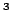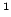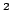Statistica Sinica 20 (2010), 1125-1142

SEMIPARAMETRIC ESTIMATION FOR REGRESSION

COEFFICIENTS IN THE COX MODEL WITH FAILURE

INDICATORS MISSING AT RANDOM

Chunling Liuand Qihua WangYunnan University,Chinese Academy of Sciences
andThe University of Hong Kong

Abstract: This paper considers a regression imputation method for estimating the regression coefficients in the Cox model when some failure indicators are missing at random, and the conditional probability of the censoring indicator is assumed to be of a parametric form. To avoid problems with missspecification of the parametric form, two augmented inverse probability weighted estimators are defined, and their asymptotic properties are established. Simulation studies were conducted to demonstrate the performance of the proposed estimators, and a data set from a stage II breast cancer trial is used to illustrate our methods.

Key words and phrases: Augmented inverse probability weighting, Cox proportional hazards model, missing at random, Nadaraya-Watson kernel estimate, regression imputation.